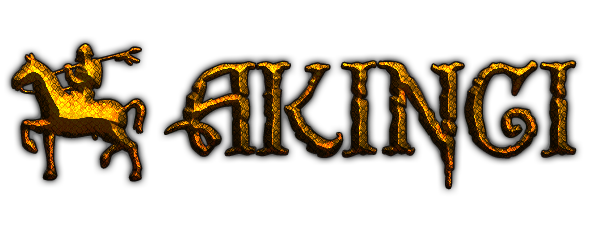The Mysterious Ancient Natarian Riddle

•Dear players,

Since the local tribe contests didn't work out as we hoped, and we didn't make it, it's time for a second try. You'll need a grasp of symbolic logic as well as lateral thinking in order to decipher the Natarian symbols into our familiar Arabic numerals. Only then will you be able to answer the following mysterious ancient Natarian riddle:

Which numbers between 0 and 9 do not form part of the following equation?

The Mysterious Ancient Natarian Riddle

Follow this link to see the equation: The ancient mysterious Natarian riddle | Travian Kingdoms Blog

• Solve the equation and replace each symbol with a number between 0 and 9
• Check which numbers are not needed to solve the equation

Assuming:

• Each achievement badge stands for a number between 0 and 9
• Each badge has its own unique number
• The three badges next to each other stand for a single number, like the number 250

Prizes:

We will give away common prizes to all players as well as individual prizes for the players who solved this mysterious riddle.

• Unlock all tribe-specific hero styles for everyone on all Travian Kingdoms worlds:

• 6 different hairstyles
• 2 new sets of eyes with a scar
• 1 new hair color
• 1 new braided beard

• 10 randomly drawn winners from among all participants with the correct answer:

• 5x 300 Gold
• 5x 100 Gold

Participation

Only one answer and avatar is allowed per participant. This means that you are also only allowed to play in one domain.

This contest runs until Monday, October 8th, at midnight CEST. We will then announce the winners here as a reply to this thread.

We will post hints here to help you find the right solution.

Good luck!

Community Manager
Travian Kingdoms International

• The answer is 4 and 9.

My avatar name is Senap and the game round I'd like the gold to is Nordic4.

Thank you for a brilliant contest! This was so much fun!

• Hello,
I do not know how to respond to it but here it is=

858 - 083 = 775
- + -
120 + 555 = 675
= = =
738 - 638 = 100

The figures not used are: 4 and 9

nickname Osha, server= tr2n

Thanks!

Numbers not used: 4 and 9

nickname: Dahee , Server: Tr2n

Thanks!

• Gaaret Com3

858 - 083 = 775
- + -
120 + 555 = 675
= = =
738 - 638 = 100

• "Which figures between 0 and 9 are not part of the following equation?"

Edit: Avatar - Akıncı
Game world - COM1The post was edited 1 time, last by Akıncı ().

• Hello there,

Numbers not used: 4 and 9

Nickname is Rick&, server is tr2n

Thank you!

• The missing numbers are 4 & 9.

Com2 Mint (Avatar for gold)

Bogus Maneuvers ®

• Hello,
The missing numbers between 0 to 9 in the equation are 4 and 9.

Equation was:
858 - 083 = 775
- + -
120 + 555 = 675
= = =
738 + 638 = 100

EDIT:
Avatar: Dormammu, World: com1x3

The post was edited 1 time, last by Dormammu.: Missed adding avatar and game world name. ().

•ATA - RAC = LLT
"""- """""""+"""""""""-
PMR + TTT = QLT
"""="""""""""=""""""""=
LCA - QCA = PRR

R = 0
P = 1
M = 2
C = 3
T = 5
Q = 6
L = 7
A = 8

NUMBERS NOT NECESSARY 4 Y 9

• The numbers which are not used are (hopefully) 4 and 9

Greetings and thanks for the Challenge• I guess the answer is 1

•  858 - 083 = 775 - + - 120 + 555 = 675 = = = 738 - 638 = 100

Spider = fish + gold (8)
brush = spider - face (5)
gold = area + fish (7)
area = face + fish (6)
hands = gold - face (2)
clock = 0
fish = 1
face = 5

Flint, com6

• I figured it out!

A (spiders) =8
B (face palm)=5
C (clock)=0
D (paint brush)=3
E (money bag)=7
F (Fish)=1
G (Hands)=2
H (squares)=6

Numbers not used: 4 and 9

The post was edited 1 time, last by PaultheGaul ().

• The numbers that are not needed to solve the equation are:
- 4 and 9
Los números que no son necesarios para resolver la ecuación son:
- 4 y 9

• The missing figures are 4 and 9.
Kuba
COM6

It took me a while